# Bohr model of Bromine atom - How to draw Bromine(Br) Bohr-Rutherford diagram?

Home  > Chemistry Article > Bromine Bohr model

The Bohr Model of Bromine(Br) has a nucleus that contains 45 neutrons and 35 protons. This nucleus is surrounded by four-electron shells named K-shell, L-shell, M-shell, and N-shell. The outermost shell in the Bohr diagram of Bromine contains 7 electrons that also called valence electrons.

 Name Bromine Bohr Model Number of neutrons 45 Number of protons 35 Number of electrons 35 Total electron shells 4 Electron in the First shell(K) 2 Electrons in the Second shell(L) 8 Electrons in the Third shell(M) 18 Electrons in the Fourth shell(N) 7 Total valence electrons in Bromine 7
Page Contents

## How to draw Bohr Model of Bromine(Br)?

Bohr model describes the visual representation of orbiting electrons around the small nucleus. It used different electron shells such as K, L, M, N…so on. These shells hold a specific number of electrons, the electron shell which is closest to the nucleus has less energy and the electron shell which is farthest from the nucleus has more energy.

Bohr diagram is very interesting and easy to draw. Here, we will draw the Bohr diagram of the Bromine atom with some simple steps.

## Steps to draw the Bohr Model of Bromine atom

1. Find the number of protons, electrons, and neutrons in the Bromine

Protons are the positively charged particles and neutrons are the uncharged particles, both these are constituents of the atom nuclei. Electrons are the negatively charged particles that orbit the nucleus of an atom

To find the number of protons an atom contains, just look at their atomic number.

If the atomic number of atom is 37, then proton will also be 37, if atomic number is 39, then proton will also be 39.

So, the atomic number for Bromine is 35, hence, the number of protons in the Bromine atom is also 35.Now, to determine the number of neutrons in an atom, use this formula.

⇒ Number of neutrons in atom = Atomic mass of the atom(rounded to the nearest whole number) – Number of proton in an atom

For example, An atom have 59.998 atomic mass and 30 protons.

Then, to find the number of neutron, round the atomic mass to the near whole number, so, atomic mass 59.998 round to 60.

= (60 – 30 protons) = 30 number of neutrons in the atom

Now, To get the number of neutrons in a Bromine atom, look at its atomic mass which is 79.904 rounded to 80, and the number of protons in Bromine is 35.

∴ Hence, the number of neutrons in Bromine atom = (80 – 35) = 45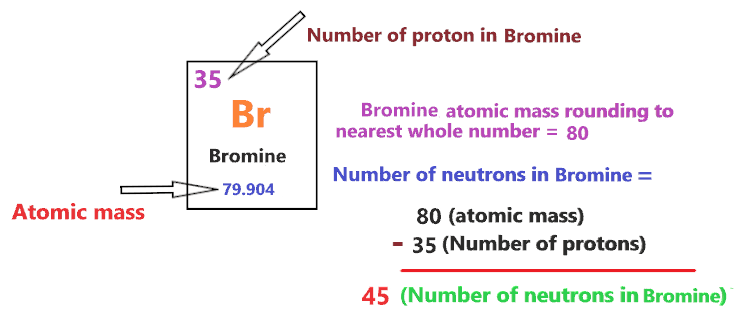It should be noted that “The number of electrons in a neutral atom is equal to the number of protons”.

So, the Bromine atom is neutral, hence, its number of electrons will be equal to the number of protons which is 35 as we already discussed.

⇒ The number of electrons in a Bromine atom = 35

⇒ The number of protons in Bromine atom = 35

⇒ The number of neutrons in a Bromine atom = 45

2. Draw the nucleus of an atom

A nucleus is a dense and small region that contains the number of protons and neutrons of an atom.

In this step, we have to draw a small circle that consists of a number of protons and the number of neutrons of a Bromine atom.3. Draw the First electron shell

“An electron shell may be thought of as an orbit followed by electrons around an atom’s nucleus.”

The first electron shell is also called the K-shell, this is the closest shell to the nucleus of an atom and can hold a maximum of two electrons.

As we know, the Bromine atom has a total of 35 electrons. So, put two electrons from it, in the first shell, next to each other.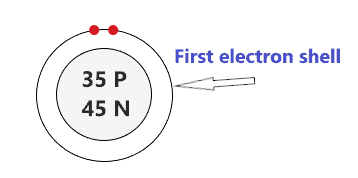We have successfully drawn the first shell of the Bromine atom that can hold 2 electrons. As a Bromine atom has a total of 35 electrons, and from 35 electrons we have used two electrons in the first shell.

∴ (35 – 2) = 33 electrons

Therefore, we are left with 33 electrons, let’s put them in the next shells of the Bromine atom.

4. Draw the Second electron shell

The second shell also called the L-shell that can hold a maximum of 8 electrons. This shell is drawn after the first electron shell.

In the second electron shell, the electrons are added one at a time, starting from the top position then going in a clockwise direction.

In second shell, electrons are added one at a time in clockwise direction as a clock position – 12 o’clock, 3 o’clock, 6 o’clock, 9 o’clock positions.Once you place the electrons one at a time to each of the four sides(Top – Right – Bottom – Left], start pairing or doubling them.

So, we have 33 remaining electrons of a Bromine atom, and the 2nd shell can only hold a maximum of 8 electrons.

Therefore, put the 8 electrons of the Bromine atom in the 2nd electron shell, start from the top position, put electrons one at a time, and go in a clockwise direction(Top – Right – Bottom – Left). And, finally, pair them up.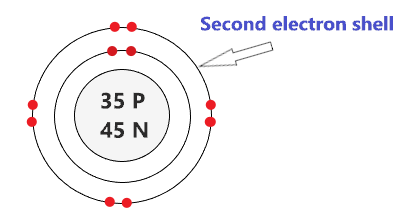As we have a total of 35 electrons for the Bromine atom and we placed 2 electrons in the first shell and 8 electrons in the second shell.

∴ [35 – (2 + 8)] = 25 electrons

hence, we are left with 25 electrons more.

Let’s place it in the next shells.

5. Draw the Third electron shell

The third shell is also called the M-shell and it has the capacity to hold up to 18 electrons but, for the elements in the first few periods, the third shell only holds up to 8 electrons.

“The third shell can be considered to hold 8 or 18 electrons but in total the third shell can hold 18 electrons.”

For the first few elements, say, till atomic number 20 on the periodic table, the third shell holds up to 8 electrons.

⇒ For atomic number 20 or less than 20, the third shell can hold up to maximum of 8 electrons.

⇒ For the atomic number more than 20, say, atomic numbers 21, 22, 23, 24, 25, 26, 27,…….etc., the third shell is filled with more than 8 electrons and up to maximum of 18 electrons.

So, we have left with 25 electrons of a Bromine atom and the third shell will hold up to 18 electrons, as the atomic number of Bromine is 35.

Therefore, put the 18 electrons of Bromine in the third shell at the position as shown in the figure below.Now after placing 18 electrons of Bromine in the third shell, we are left with only 7 electrons.

Let’s put them in the next shell.

6. Draw the Fourth electron shell

Here, draw the fourth electron shell and put the remaining 7 electrons of Bromine in such a way that, start from the top position, put electrons one at a time, and go in a clockwise direction(Top – Right – Bottom – Left). And, finally, start pairing.### Bohr model of the Bromine atom

That’s all, this is our Bohr model of the Bromine atom that contains 35 protons and 45 neutrons in the nucleus region, and 35 electrons are orbited around the nucleus, two electrons in the first shell, eight electrons in the second shell, eighteen electrons in the third shell, and seven electrons in the fourth shell.

## Find Valence electron of Bromine through its Bohr diagram

From the Bohr diagram of an atom, we can easily find the number of valence electrons in an atom by looking at its outermost shell.

So, we have to find a valence electron in the Bromine atom, for this, look at its Bohr diagram.

Bohr’s diagram of Bromine has four electron shells (K, L, M, N), the inner shell is K-shell and the outermost shell is N-shell.

Hence, the electrons found in the N-shell of the Bromine atom are its valence electrons because it is the outermost shell that also called the valence shell.

The N-shell or outer shell of the Bromine Bohr model contains 7 electrons, therefore, the number of the valence electrons in the Bromine atom is also 7.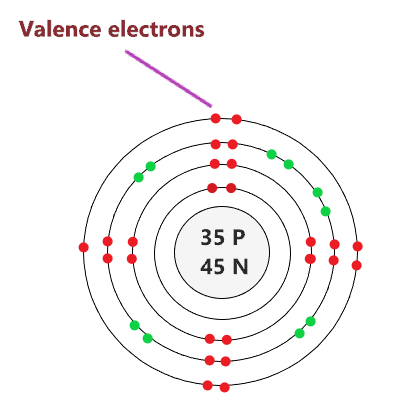## Electron dot diagram of a Bromine atom

Electron dot diagram also called lewis structure which represents the valence electrons of atoms.

As, from the Bohr diagram of Bromine, we got to know, it has 7 valence electrons.

So, just represent the 7 valence electron around the Bromine atom as a dot.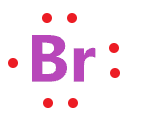## The electron configuration of Bromine

Bromine has an atomic number of 35 and it contains a total number of 35 electrons. From the Bohr model of Bromine, we know, 2 electrons are in the K-shell, 8 electrons are in the L-shell, 18 electrons are in the M-shell and 7 electrons are in the N-shell.

So, based on the shell, the electronic configuration of the Bromine atom is [2, 8, 18, 7].

Or the electronic configuration of Bromine is [Ar] 3d¹⁰ 4s² 4p⁵ since it contains a total of 35 electrons.

## FAQ

### How many electron shells a Bromine Bohr model contains?

Electron shell also called energy level, you can find the number of electron shells for an element by knowing its period number in the periodic table.

The elements or atoms in the first period of the periodic table have one energy level or one electron shell, same as, the elements in the second period have two energy levels or two-electron shells and so on…

So, the Bromine atom belongs to the 4th Period in the periodic table, hence, the number of electron shells for the Bohr model of Bromine will be 4 (K-shell, L-shell, M-shell, and N-shell).

### What is the outer shell of the Bohr diagram of the Bromine atom?

The outermost shell also called the valence shell, which contains valence electrons of the atom.

According to the Bohr diagram of Bromine, the outer shell is N-shell which contains seven valence electrons.

## Properties of Bromine

1. Bromine is the third-lightest halogen.
2. It is very reactive in nature.
3. It appears as reddish-brown.
4. It is one electron short of a full octet.
5. It forms an orthorhombic crystal structure.

## Summary

• The Bohr model of Bromine(Br) is drawn with four electron shells, the first shell contains 2 electrons, the second shell contains 8 electrons, the third shell contains 18 electrons and the fourth shell contains 7 electrons.
• Bromine is neutral and its atomic number is 35, hence, the number of protons and electrons available for its Bohr diagram is also 35.
• The number of neutrons for the Bohr diagram of Bromine can be found by subtracting the number of protons from the atomic mass(rounded to the nearest whole).
• The electron configuration of Bromine in terms of the shell is [2, 8, 18, 7], or in normal form, it is [Ar] 3d¹⁰ 4s² 4p⁵.
##### Subscribe to Blog via Email

Join 2 other subscribers

Share it...